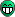Page 1 of 1

### Max. mA for IO-Pin?

Posted: Sun Jul 09, 2017 4:04 pm
What is the max. mA for a GPIO-Pin of the esp32? I read something about 40mA, but couldn't find it in the datasheet.

### Re: Max. mA for IO-Pin?

Posted: Sun Jul 09, 2017 4:24 pm
based on this thread, it appears that the maximum one can safely draw from a GPIO pin is 12ma.

https://esp32.com/viewtopic.php?f=2&t=2027

### Re: Max. mA for IO-Pin?

Posted: Sun Jul 09, 2017 4:45 pm
And how much ampere goes threw a gpio when i put it high? So i can calculate what resistors i need.

### Re: Max. mA for IO-Pin?

Posted: Sun Jul 09, 2017 5:21 pm
My understanding is that electrical current is a movement of electrons measures in Amperes. A GPIO pin exposes a signal level that is either 3.3V or 0V. The maximum amount of current that you can allow to flow out of the GPIO pin is 12mA.

The GPIO pin is a "source" of current flow but there will be something you connect to the GPIO which will be a "sink" (consumer) of current. What I think we are saying in this story is that you must constrain the sink to take no more current than 12mA from the GPIO. Without knowing more about what it connecting to the GPIO and how much current it needs to operate/trigger, I don't think we have enough information to assist you with an answer.

### Re: Max. mA for IO-Pin?

Posted: Sun Jul 09, 2017 5:52 pm
Saskia wrote:And how much ampere goes threw a gpio when i put it high? So i can calculate what resistors i need.

We have 3.3 V as U

We have 12 mA for I

you need R

R = 3.3V / 0.012 A

R = 275 Ohm

so if you use 275 Ohm as R and we calculate 3.3V
you are on secure side, that you not consum more as 12mA

--------------------------------------------------------------------------

if there is 40mA

R = 3.3V / 0.04 A

R = 82.5 Ohm

--------------------------------------------------------------------------

hope this helps

this is only for "Unbranched circuit"

if you want connect a LED to GPIO

the LED has 2.2V and need 20 mA and you use 275 Ohm as Resistor
the Led power is driven by 0.6 faktor ( 12 : 20 )

hope this helps

best wishes
rudiedit: what you search for is "ohmic law"

edit: if you want drive the led 100 procent
( you need more as 12 mA on Pin )

you have
U = 3.3V
U led = 2.2V
I led = 20mA

then you must calculate U resistor, then you can calculate R led

U = U led + U resistor
U resistor = U - U led
U resistor = 3.3V - 2.2V
U resistor = 1.1V

Resistor = U resistor / I
Resistor = 1.1V / 0.02 A ( I led )
Resistor = 55 Ohm

what you search for is "Resistance , Current limiting resistor"

Led Power on 12 mA / 20 mA

P = U * I
so

12mA
P = U led * I led
P = 2.2 * 0.012
P = 0,0264 Watt

20mA
P = U led * I led
P = 2.2 * 0.02
P = 0,044

with 12 mA you drive your LED on factor ( procent ) :

P 12mA = 0.0264 W
P 20mA = 0.044 W

P procent = ( P 12mA : P 20mA ) * 100
P procent = 0,6 * 100
P procent = 60 %

edit: and last but not least

if you have 40 mA on Pin
you must use a Resistor for limiting current
so use then the 55 Ohm resistor in the line and you will drive the LED on 2.2V and a max current of 20mA.
if there is a limit from GPIO on current example 12mA you will drive the Led with a maximum of 60 procent power.

### Re: Max. mA for IO-Pin?

Posted: Sun Jul 09, 2017 6:24 pm
Oh, well. That was a stupid question. Thanks anyway!

### Re: Max. mA for IO-Pin?

Posted: Sun Jul 09, 2017 6:36 pm
Saskia wrote:Oh, well. That was a stupid question. Thanks anyway!
you're welcomeno question is stupid enougthat you not can get an answerebe sure, there are different LED on the market, and each color need other voltage too
it is not a general saying with 2.2 V and not a general saying that red, green, yellow or blue need the same voltage
it is allways different.

you must read the Voltage need of your consum hardware from the datasheet.
and the sink of Ampere - usually is this in milli Ampere
what is 1 / 1000 .
1000 mA = 1 A

hope this helps
best wishes
rudi### Re: Max. mA for IO-Pin?

Posted: Mon Jul 10, 2017 5:11 am
Doesn't the internal resistance of the current source also play role? Basically the impedance of the GPIO pin? Not that it matters, just curious.